Courses

# Test: 35 Year JEE Previous Year Questions: The Solid State & Surface Chemistry

## 26 Questions MCQ Test Class 12 Chemistry 35 Years JEE Mains &Advance Past yr Paper | Test: 35 Year JEE Previous Year Questions: The Solid State & Surface Chemistry

Description
This mock test of Test: 35 Year JEE Previous Year Questions: The Solid State & Surface Chemistry for JEE helps you for every JEE entrance exam. This contains 26 Multiple Choice Questions for JEE Test: 35 Year JEE Previous Year Questions: The Solid State & Surface Chemistry (mcq) to study with solutions a complete question bank. The solved questions answers in this Test: 35 Year JEE Previous Year Questions: The Solid State & Surface Chemistry quiz give you a good mix of easy questions and tough questions. JEE students definitely take this Test: 35 Year JEE Previous Year Questions: The Solid State & Surface Chemistry exercise for a better result in the exam. You can find other Test: 35 Year JEE Previous Year Questions: The Solid State & Surface Chemistry extra questions, long questions & short questions for JEE on EduRev as well by searching above.
QUESTION: 1

### The formation of gas at the surface of tungsten due toadsorption is the reaction of order        

Solution:

It is zero order reaction
[NOTE : Adsorption of gas on metal surface is of zero
order]

QUESTION: 2

### Na and Mg crystallize in BCC and FCC type crystalsrespectively, then the number of atoms of Na and Mg presentin the unit cell of their respective crystal is                   

Solution:

In bcc - points are at corners and one in the centre of the unit cell.
Number of atoms per unit cell =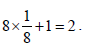In fcc - points are at the corners and also centre of the six faces of each cell.
Number of atoms per unit cell =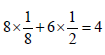QUESTION: 3

### How many unit cells are present in a cube-shaped idealcrystal of NaCl of mass 1.00 g ?                    [Atomic masses : Na = 23, Cl = 35.5]

Solution:

Since in NaCl type of structure 4 formula units form a cell. Number of formulas in cube shaped crystals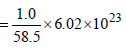No. of unit cells present in a cubic crystal =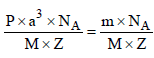∴ units cells =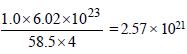unit cells.

QUESTION: 4

Which one of the following characteristics is not correct forphysical adsorption ?            

Solution:

As adsorption is an exothermic process.
∴ Rise in temperature will decrease adsorption
(according to Le-chatelier principle).

QUESTION: 5

What type of crystal defect is indicated in the diagram below?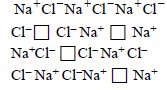Solution:

When equal number of cations and anions are missing from their regular lattice positions, we have schottky defect.
This type of defects are more common in ionic
compounds with high co-ordination number and where the size of positive and negative ions are almost equal
e.g. NaCl KCl etc.

QUESTION: 6

Identify the correct statement regarding enzymes 

Solution:

Enzymes are very specific biological catalysts
possessing well - defined active sites

QUESTION: 7

An ionic compound has a unit cell consisting of A ions atthe corners of a cube and B ions on the centres of the facesof the cube. The empirical formula for this compound wouldbe 

Solution:

Number of A ions in the unit cell =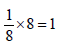Number of B ions in the unit cell =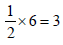Hence empirical formula of the compound = AB3

QUESTION: 8

The volume of a colloidal particle, VC as compared to the volume of a solute particle in a true solution VS , could be

Solution:

Particle size of colloidal particle = 1mμ to 100 mμ      (suppose 10 mμ)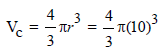Particle size of true solution particle = 1mm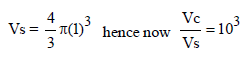QUESTION: 9

The disperse phase in colloidal iron (III) hydroxide andcolloidal gold is positively and negatively charged,respectively. Which of the following statements is NOTcorrect ?            

Solution:

When oppositely charged sols are mixed their charges are neutralised. Both sols may be partially or completely precipitated.

QUESTION: 10

Total volume of atoms present in a face-centred cubic unit cell of a metal is (r is atomic radius) 

Solution:

The face centered cubic unit cell contains 4 atom
∴ Total volume of atoms =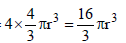QUESTION: 11

In Langmuir's model of adsorption of a gas on a solid surface

Solution:

According to Langmuir's Model of adsorption of a gas on a soild surface the mass of gas adsorbed(x)per gram of the adsorbent (m) is directly proportional to the pressure of the gas (p) at constant temperature i.e.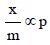QUESTION: 12

In a compound, atoms of element Y form ccp lattice andthose of element X occupy 2/3rd of tetrahedral voids. Theformula of the compound will be 

Solution:

From the given data, we have
Number of Y atoms in a unit cell = 4
Number of X atoms in a unit cell =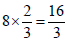From the above we get the formula of the compound as
X16 / 3 Y4 or X4Y3

QUESTION: 13

Gold numbers of protective colloids A, B, C and D are 0.50,0.01, 0.10 amd 0.005, respectively. the correct order of theirprotective powers is       

Solution:

For a protective colloid μ lesser the value of gold number better is the protective power.
Thus the correct order of protective power of A, B, C and D is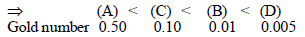Hence (c) is the correct answer

QUESTION: 14

Which of the following statements is incorrect regardingphysissorptions? 

Solution:

Adsorption is an exothermic process, hence DH will always be negative

QUESTION: 15

Copper crystallises in fcc with a unit cell length of 361 pm.What is the radius of copper atom? 

Solution:

For fcc unit cell,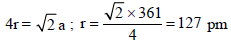QUESTION: 16

The edge length of a face centered cubic cell of an ionicsubstance is 508 pm. If the radius of the cation is 110 pm, theradius of the anion is             

Solution:

For an Fcc crystal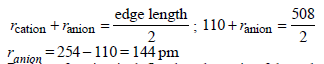QUESTION: 17

Percentages of free space in cubic close packed structureand in body centered packed structure are respectively

Solution:

Packing fraction is defined as the ratio of the volume of the unit cell that is occupied by the spheres to the volume of the unit cell.
P.F. for cpp and bcc are 0.74 and 0.68 respectively.
So, the free space in ccp and bcc are 26% & 32% respectively.

QUESTION: 18

In a face centred cubic lattice, atom A occupies the cornerpositions and atom B occupies the face centre positions. Ifone atom of B is missing from one of the face centred points,the formula of the compound is : 

Solution:

No. of atoms in the corners (A)  =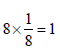No. of atoms at face centres (B) =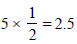∴ Formula is = AB2.5 or A2B5

QUESTION: 19

Lithium forms body centred cubic structure. The length ofthe side of its unit cell is 351 pm. Atomic radius of the lithiumwill be :                                  

Solution:

For BCC structure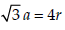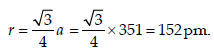QUESTION: 20

According to Freundlich adsorption isotherm which of the following is correct?                      

Solution:

The Freundlich adsorption isotherm is mathematically represented as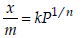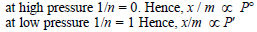QUESTION: 21

Which of the following exists as covalent crystals in thesolid state ?               [JEE M 2013]

Solution:

Among the given crystals only silicon is as a covalent solid.

QUESTION: 22

The coagulating power of electrolytes having ions Na+, Al3+and Ba2+ for arsenic sulphide sol increases in the order :         [JEE M 2013]

Solution:

According to Hardly Schulze rule, greater the charge on cation, greater is its coagulating power for negatively charged solution. hence the correct order of coagulating power : Na+ < Ba2+ < Al3+

QUESTION: 23

CsCl crystallises in body centred cubic lattice. If ‘a’ is its edge length then which of the following expressions is
correct?

Solution: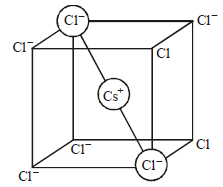Relation between radius of cation, anion and edge length of the cube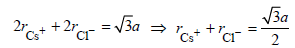QUESTION: 24

Sodium metal crystallizes in a body centred cubic lattice witha unit cell edge of 4.29Å. The radius of sodium atom isapproximately :               [JEE M 2015]

Solution:

In bcc the atoms touch along body diagonal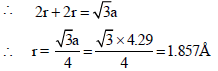QUESTION: 25

3 g of activated charcoal was added to 50 mL of acetic acidsolution (0.06N) in a flask. After an hour it was filtered andthe strength of the filtrate was found to be 0.042 N. Theamount of acetic acid adsorbed (per gram of charcoal) is :            [JEE M 2015]

Solution:

Let the weight of acetic acid initially be w1 in 50 ml of 0.060 N solution

Let the N =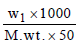(Normality = 0.06 N)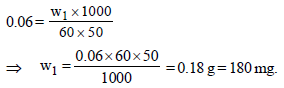After an hour, the strength of acetic acid = 0.042 N
so, let the weight of acetic acid be w2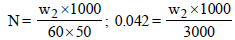⇒ w2 = 0.126 g = 126 mg
So amount of acetic acid adsorbed per 3g
= 180 – 126 mg = 54 mg
Amount of acetic acid adsorbed per g
= 54/3 = 18 mg

QUESTION: 26

For a linear plot of log (x/m) versus log p in a Freundlichadsorption isotherm, which of the following statements iscorrect? (k and n are constants) [JEE M 2016]

Solution:

According to Freundlich adsorption isotherm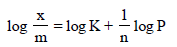Thus if a graph is plotted between log (x/m) and log P, a straight line will be obtained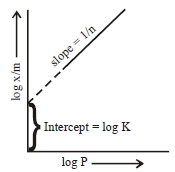The slope of the line is equal to 1/n and the intercept on log x/m axis will correspond to log K.